# bubblechart

•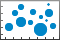## Syntax

``bubblechart(x,y,sz)``
``bubblechart(x,y,sz,c)``
``bubblechart(tbl,xvar,yvar,sizevar)``
``bubblechart(tbl,xvar,yvar,sizevar,cvar)``
``bubblechart(ax,___)``
``bubblechart(___,Name,Value)``
``bc = bubblechart(___)``

## Description

### Vector and Matrix Data

example

````bubblechart(x,y,sz)` displays colored circular markers (bubbles) at the locations specified by the vectors `x` and `y`, with bubble sizes specified by `sz`. To plot one set of coordinates, specify `x`, `y`, and `sz` as vectors of equal length.To plot multiple sets of coordinates on the same set of axes, specify at least one of `x`, `y`, or `sz` as a matrix. ```

example

````bubblechart(x,y,sz,c)` specifies the colors of the bubbles. You can specify one color for all the bubbles, or you can vary the color. For example, you can plot all red bubbles by specifying `c` as `"red"`.```

### Table Data

example

````bubblechart(tbl,xvar,yvar,sizevar)` plots the variables `xvar` and `yvar` from the table `tbl`, and uses the variable `sizevar` for the bubble sizes. To plot one data set, specify one variable each for `xvar`, `yvar`, and `sizevar`. To plot multiple data sets, specify multiple variables for at least one of those arguments. The arguments that specify multiple variables must specify the same number of variables.```

example

````bubblechart(tbl,xvar,yvar,sizevar,cvar)` plots the specified variables from the table using the colors specified in the variable `cvar`. To specify colors for multiple data sets, specify `cvar` as multiple variables. The number of variables must match the number of data sets.```

example

````bubblechart(ax,___)` displays the bubble chart in the target axes `ax`. Specify the axes before all other input arguments.```

example

````bubblechart(___,Name,Value)` specifies `BubbleChart` properties using one or more name-value arguments. Specify the properties after all other input arguments. For a list of properties, see BubbleChart Properties.```

example

````bc = bubblechart(___)` returns the `BubbleChart` object. Use `bc` to modify properties of the chart after creating it. For a list of properties, see BubbleChart Properties.```

## Examples

collapse all

Define the bubble coordinates as the vectors `x` and `y`. Define `sz` as a vector that specifies the bubble sizes. Then create a bubble chart of `x` and `y`.

```x = 1:20; y = rand(1,20); sz = rand(1,20); bubblechart(x,y,sz);```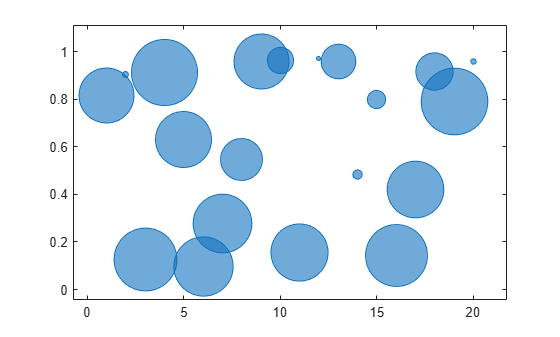Define the bubble coordinates as the vectors `x` and `y`. Define `sz` as a vector that specifies the bubble sizes. Then create a bubble chart of `x` and `y`, and specify the color as red. By default, the bubbles are partially transparent.

```x = 1:20; y = rand(1,20); sz = rand(1,20); bubblechart(x,y,sz,'red');```For a custom color, you can specify an RGB triplet or a hexadecimal color code. For example, the hexadecimal color code `'#7031BB'` specifies a shade of purple.

`bubblechart(x,y,sz,'#7031BB');`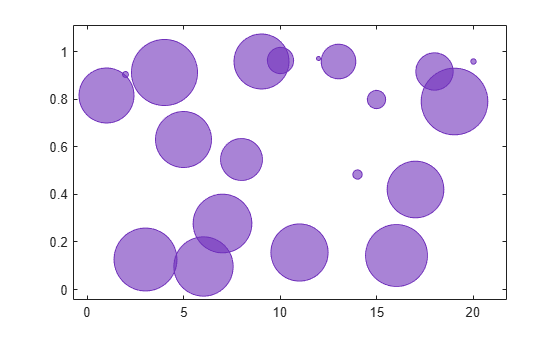You can also specify a different color for each bubble. For example, specify a vector to select colors from the figure's colormap.

```c = 1:20; bubblechart(x,y,sz,c)```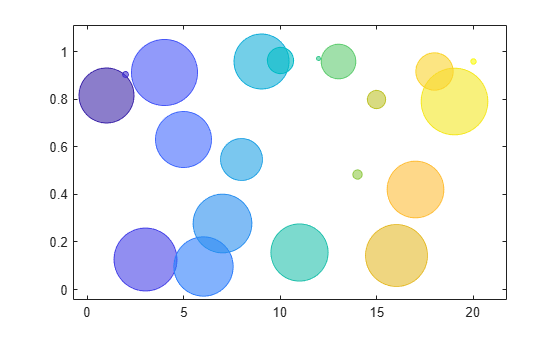Define the bubble coordinates as the vectors `x` and `y`. Define `sz` as a vector that specifies the bubble sizes. Then create a bubble chart of `x` and `y`. By default, the bubbles are 60% opaque, and the edges are completely opaque with the same color.

```x = 1:20; y = rand(1,20); sz = rand(1,20); bubblechart(x,y,sz);```You can customize the opacity and the outline color by setting the `MarkerFaceAlpha` and `MarkerEdgeColor` properties, respectively. One way to set a property is by specifying a name-value pair argument when you create the chart. For example, you can specify 20% opacity by setting the `MarkerFaceAlpha` value to `0.20`.

`bc = bubblechart(x,y,sz,'MarkerFaceAlpha',0.20);`If you create the chart by calling the `bubblechart` function with a return argument, you can use the return argument to set properties on the chart after creating it. For example, you can change the outline color to purple.

`bc.MarkerEdgeColor = [0.5 0 0.5];`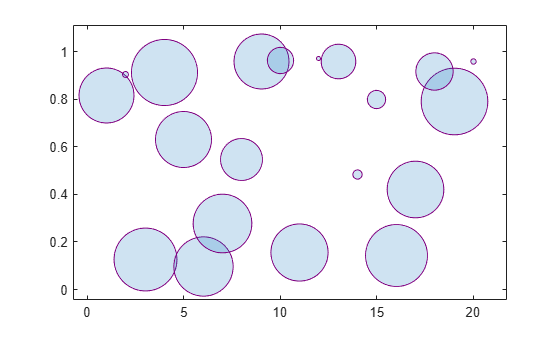Define a data set that shows the contamination levels of a certain toxin across different towns in a metropolitan area. Define `towns` as the population of each town. Define `nsites` as the number of industrial sites in the corresponding towns. Define `levels` as the contamination levels in the towns. Then display the data in a bubble chart with axis labels. Call the `bubblesize` function to decrease the bubble sizes, and add a bubble legend that shows the relationship between the bubble size and population.

```towns = randi([25000 500000],[1 30]); nsites = randi(10,1,30); levels = (3 * nsites) + (7 * randn(1,30) + 20); % Display bubble chart with axis labels and legend bubblechart(nsites,levels,towns) xlabel('Number of Industrial Sites') ylabel('Contamination Level') bubblesize([5 30]) bubblelegend('Town Population','Location','eastoutside')```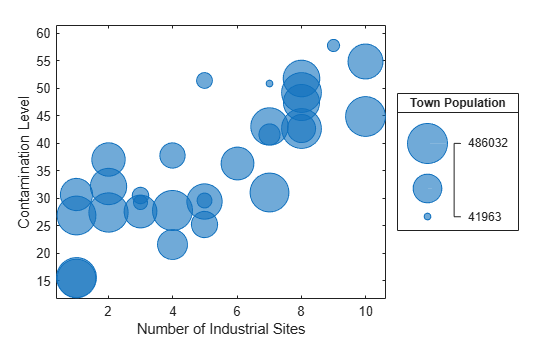When you display multiple data sets in the same axes, you can include multiple legends. To manage the alignment of the legends, create your chart in a tiled chart layout.

Create two sets of data, and plot them together in the same axes object within a tiled chart layout.

```x = 1:20; y1 = rand(1,20); y2 = rand(1,20); sz1 = randi([20 500],[1,20]); sz2 = randi([20 500],[1,20]); % Plot data in a tiled chart layout t = tiledlayout(1,1); nexttile bubblechart(x,y1,sz1) hold on bubblechart(x,y2,sz1) hold off```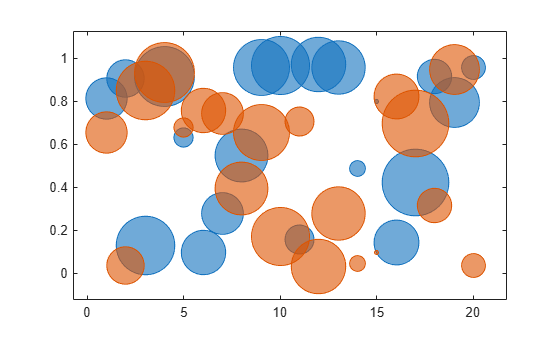Add a bubble legend for illustrating the bubble sizes, and add another legend for illustrating the colors. Call the `bubblelegend` and `legend` functions with a return argument to store each legend object. Move the legends to the right outer tile of the tiled chart layout by setting the `Layout.Tile` property on each object to `'east'`.

```blgd = bubblelegend('Population'); lgd = legend('Springfield','Fairview'); blgd.Layout.Tile = 'east'; lgd.Layout.Tile = 'east';```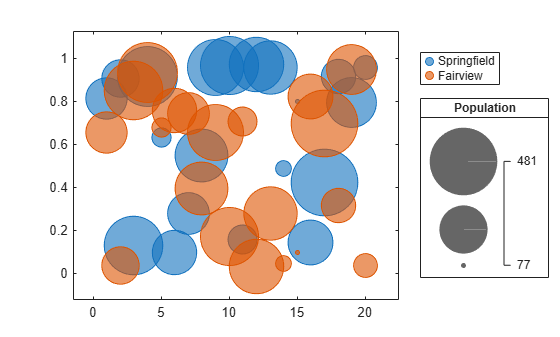A convenient way to plot data from a table is to pass the table to the `bubblechart` function and specify the variables you want to plot. For example, read `patients.xls` as a table `tbl`. Plot the `Systolic`, `Diastolic`, and `Weight` variables by passing `tbl` as the first argument to the `bubblechart` function followed by the variable names. By default, the axis labels match the variable names.

```tbl = readtable('patients.xls'); bubblechart(tbl,'Systolic','Diastolic','Weight');```You can also plot multiple variables at the same time. For example, plot both blood pressure variables versus the `Height` variable by specifying the `yvar` argument as the cell array `{'Systolic','Diastolic'}`. Change the range of bubble sizes to be between `5` and `20` points. Then add a legend. The legend labels match the variable names.

```bubblechart(tbl,'Height',{'Systolic','Diastolic'},'Weight'); bubblesize([5 20]) legend```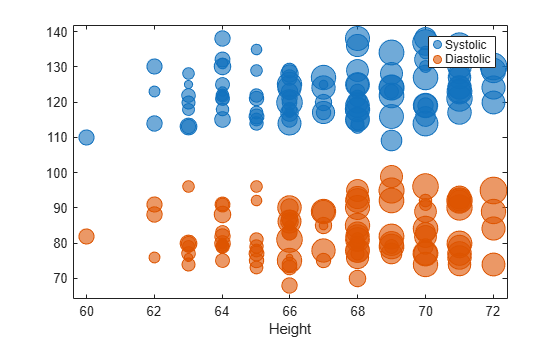You can plot data from a table and customize the colors by specifying the `cvar` argument when you call `bubblechart`.

For example, create a table with four variables of random numbers, and plot the `X` `and` `Y` variables. Vary the bubble sizes according to the `Sz` variable, and vary the colors according to the `Colors` variable.

```tbl = table(randn(15,1)-10,randn(15,1)+10,rand(15,1),rand(15,1), ... 'VariableNames',{'X','Y','Sz','Colors'}); bubblechart(tbl,'X','Y','Sz','Colors');```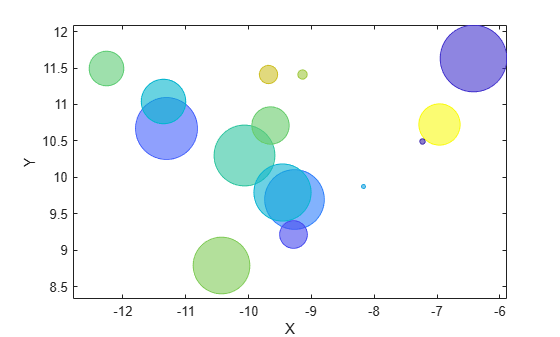Define two sets of data that show the contamination levels of a certain toxin across different towns on the east and west sides of a certain metropolitan area. Define `towns1` and `towns2` as the populations across the towns. Define `nsites1` and `nsites2` as the number of industrial sites in the corresponding towns. Then define `levels1` and `levels2` as the contamination levels in the towns.

```towns1 = randi([25000 500000],[1 30]); towns2 = towns1/3; nsites1 = randi(10,1,30); nsites2 = randi(10,1,30); levels1 = (5 * nsites2) + (7 * randn(1,30) + 20); levels2 = (3 * nsites1) + (7 * randn(1,30) + 20);```

Create a tiled chart layout so you can visualize the data side-by-side. Then create an axes object in the first tile and plot the data for the west side of the city. Add a title and axis labels. Then, repeat the process in the second tile to plot the east side data.

```tiledlayout(1,2,'TileSpacing','compact') % West side ax1 = nexttile; bubblechart(ax1,nsites1,levels1,towns1); title('West Side') xlabel('Number of Industrial Sites') % East side ax2 = nexttile; bubblechart(ax2,nsites2,levels2,towns2); title('East Side') xlabel('Number of Industrial Sites') ylabel('Contamination Level')```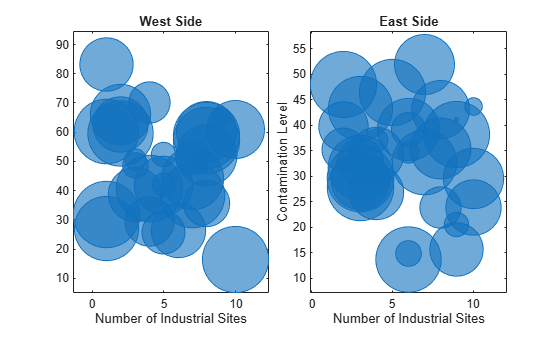Reduce all the bubble sizes to make it easier to see all the bubbles. In this case, change the range of diameters to be between `5` and `30` points.

```bubblesize(ax1,[5 30]) bubblesize(ax2,[5 30])```The west side towns are three times the size of the east side towns, but the bubble sizes do not reflect this information in the preceding charts. This is because the smallest and largest bubbles map to the smallest and largest data points in each of the axes. To display the bubbles on the same scale, define a vector called `alltowns` that includes the populations from both sides of the city. Use the `bubblelim` function to reset the scaling for both charts. Next, use the `xlim` and `ylim` functions to display the charts with the same x- and y-axis limits.

```% Adjust scale of the bubbles alltowns = [towns1 towns2]; newlims = [min(alltowns) max(alltowns)]; bubblelim(ax1,newlims) bubblelim(ax2,newlims) % Adjust x-axis limits allx = [xlim(ax1) xlim(ax2)]; xmin = min(allx); xmax = max(allx); xlim([ax1 ax2],[xmin xmax]); % Adjust y-axis limits ally = [ylim(ax1) ylim(ax2)]; ymin = min(ally); ymax = max(ally); ylim([ax1 ax2],[ymin ymax]);```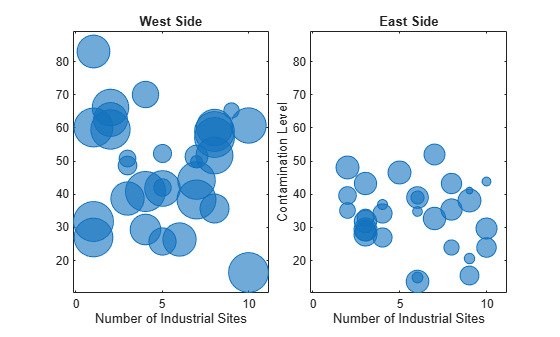## Input Arguments

collapse all

x-coordinates, specified as a scalar, vector, or matrix. The size and shape of `x` depends on the shape of your data. This table describes the most common situations.

Type of Bubble ChartHow to Specify Coordinates
Single bubble

Specify `x`, `y`, and `sz` as scalars. For example:

`bubblechart(1,2,10)`

One set of bubbles

Specify `x`, `y`, and `sz` as any combination of row or column vectors of the same length. For example:

```x = [1 2 3 4]; y = [4; 5; 6; 7]; sz = [12 13 14 15]; bubblechart(x,y,sz)```

Multiple sets of bubbles

If all the data sets share the same x- or y-coordinates, specify the shared coordinates as a vector and the other coordinates as a matrix. The length of the vector must match one of the dimensions of the matrix. For example, plot two data sets that share the same x-coordinates and size values.

```x = [1 2 3 4]; y = [4 5 6 7; 7 8 9 10]; sz = [1 2 3 4]; bubblechart(x,y,sz)```
If the matrices are square, `bubblechart` plots a separate set of bubbles for each column in the matrix.

Alternatively, specify `x`, `y`, and `sz` as matrices of equal size. In this case, `bubblechart` plots the columns of the matrices. For example, plot two data sets.

```x = [1 1; 2 2; 3 3; 4 4]; y = [4 7; 5 8; 6 9; 7 10]; sz = [1 1; 2 2; 3 3; 4 4]; bubblechart(x,y,sz)```

Data Types: `single` | `double` | `int8` | `int16` | `int32` | `int64` | `uint8` | `uint16` | `uint32` | `uint64` | `categorical` | `datetime` | `duration`

y-coordinates, specified as a scalar, vector, or matrix. The size and shape of `y` depends on the shape of your data. This table describes the most common situations.

Type of Bubble ChartHow to Specify Coordinates
Single bubble

Specify `x`, `y`, and `sz` as scalars. For example:

`bubblechart(1,2,10)`

One set of bubbles

Specify `x`, `y`, and `sz` as any combination of row or column vectors of the same length. For example:

```x = [1 2 3 4]; y = [4; 5; 6; 7]; sz = [12 13 14 15]; bubblechart(x,y,sz)```

Multiple sets of bubbles

If all the data sets share the same x- or y-coordinates, specify the shared coordinates as a vector and the other coordinates as a matrix. The length of the vector must match one of the dimensions of the matrix. For example, plot two data sets that share the same x-coordinates and size values.

```x = [1 2 3 4]; y = [4 5 6 7; 7 8 9 10]; sz = [1 2 3 4]; bubblechart(x,y,sz)```
If the matrices are square, `bubblechart` plots a separate set of bubbles for each column in the matrix.

Alternatively, specify `x`, `y`, and `sz` as matrices of equal size. In this case, `bubblechart` plots the columns of the matrices. For example, plot two data sets.

```x = [1 1; 2 2; 3 3; 4 4]; y = [4 7; 5 8; 6 9; 7 10]; sz = [1 1; 2 2; 3 3; 4 4]; bubblechart(x,y,sz)```

Data Types: `single` | `double` | `int8` | `int16` | `int32` | `int64` | `uint8` | `uint16` | `uint32` | `uint64` | `categorical` | `datetime` | `duration`

Relative bubble sizes, specified as a numeric scalar, vector, or matrix.

The `sz` values control the relative distribution of the bubble sizes. By default, `bubblechart` linearly maps a range of bubble areas across the range of the `sz` values for all the bubble charts in the axes. For more control over the absolute bubble sizes, and how they map across the range of the `sz` values, see `bubblesize` and `bubblelim`.

Whether you specify `sz` as a scalar, vector, or matrix depends on how you specify `x` and `y` and how you want the chart to look. This table describes the most common situations.

Type of Bubble Chart`x` and `y` `sz`Example

One set of bubbles

Vectors of the same length

A vector with the same length as `x` and `y`

Specify `x`, `y`, and `sz` as vectors.

```x = [1 2 3 4]; y = [4 5 6 7]; sz = [80 150 700 50]; bubblechart(x,y,sz)```

Multiple sets of bubbles that have varied coordinates and bubble sizes

At least one of `x` or `y` is a matrix for plotting multiple data sets

A matrix that has the same size as the `x` or `y` matrix

Specify `x` as a vector and `y` and `sz` as matrices.

```x = [1 2 3 4]; y = [1 6; 3 8; 2 7; 4 9]; sz = [80 30; 150 900; 50 2000; 200 350]; bubblechart(x,y,sz)```

Multiple sets of bubbles, where all the coordinates are shared, but the sizes are different for each data set

Vectors of the same length

A matrix with at least one dimension that matches the lengths of `x` and `y`

Specify `x` and `y` as vectors and `sz` as a matrix.

```x = [1 2 3 4]; y = [5 6 7 8]; sz = [80 30; 150 900; 50 500; 200 350]; bubblechart(x,y,sz)```

Multiple sets of bubbles, where the coordinates vary in at least one dimension, but the sizes are shared between data sets

At least one of `x` or `y` is a matrix for plotting multiple data sets

A vector with the same number of elements as there are bubbles in each data set

Specify `x` as a vector, `y` as a matrix, and `sz` as vector.

```x = [1 2 3 4]; y = [1 6; 3 8; 2 7; 4 9]; sz = [80 150 200 350]; bubblechart(x,y,sz)```

Data Types: `single` | `double` | `int8` | `int16` | `int32` | `int64` | `uint8` | `uint16` | `uint32` | `uint64`

Bubble color, specified as a color name, RGB triplet, matrix of RGB triplets, or a vector of colormap indices.

• Color name — A color name such as `"red"`, or a short name such as `"r"`.

• RGB triplet — A three-element row vector whose elements specify the intensities of the red, green, and blue components of the color. The intensities must be in the range `[0,1]`; for example, ```[0.4 0.6 0.7]```. RGB triplets are useful for creating custom colors.

• Matrix of RGB triplets — A three-column matrix in which each row is an RGB triplet.

• Vector of colormap indices — A vector of numeric values that is the same length as the `x` and `y` vectors.

The way you specify the color depends on your preferred color scheme and whether you are plotting one set of bubbles or multiple sets of bubbles. This table describes the most common situations.

Color SchemeHow to Specify the ColorExample

Use one color for all the bubbles.

Specify a color name or a short name from the table below, or specify one RGB triplet.

Display one set of bubbles, and specify the color as `"red"`.

```x = [1 2 3 4]; y = [2 5 3 6]; sz = [1 2 3 4]; bubblechart(x,y,sz,"red")```

Display two sets of bubbles, and specify the color as red using the RGB triplet ```[1 0 0]```.

```x = [1 2 3 4]; y = [2 5; 1 2; 8 4; 7 9]; sz = [1 2; 3 4; 5 6; 7 8]; bubblechart(x,y,sz,[1 0 0])```

Assign different colors to each bubble using a colormap.

Specify a row or column vector of numbers. The numbers map into the current colormap array. The smallest value maps to the first row in the colormap, and the largest value maps to the last row. The intermediate values map linearly to the intermediate rows.

If your chart has three bubbles, specify a column vector to ensure the values are interpreted as colormap indices.

You can use this method only when `x`, `y`, and `sz` are all vectors.

Create a vector `c` that specifies four colormap indices. Display four bubbles using the colors from the current colormap. Then, change the colormap to `winter`.

```c = [1 2 3 4]; x = [1 2 3 4]; y = [5 6 7 8]; sz = [1 2 3 4]; bubblechart(x,y,sz,c) colormap(gca,"winter")```

Create a custom color for each bubble.

Specify an m-by-3 matrix of RGB triplets, where m is the number of bubbles.

You can use this method only when `x`, `y`, and `sz` are all vectors.

Create a matrix `c` that specifies RGB triplets for green, red, gray, and purple. Then display four bubbles using those colors.

```c = [0 1 0; 1 0 0; 0.5 0.5 0.5; 0.6 0 1]; x = [1 2 3 4]; y = [5 6 7 8]; sz = [1 2 3 4]; bubblechart(x,y,sz,c)```

Create a different color for each data set.

Specify an n-by-3 matrix of RGB triplets, where n is the number of data sets.

You can use this method only when at least one of `x`, `y`, or `sz` is a matrix.

Create a matrix `c` that contains two RGB triplets. Then display two sets of bubbles using those colors.

```c = [1 0 0; 0.6 0 1]; x = [1 2 3 4]; y = [2 5; 1 2; 8 4; 11 9]; sz = [1 1; 2 2; 3 3; 4 4]; bubblechart(x,y,sz,c) ```

#### Color Names and RGB Triplets for Common Colors

Color NameShort NameRGB TripletHexadecimal Color CodeAppearance
`"red"``"r"``[1 0 0]``"#FF0000"``"green"``"g"``[0 1 0]``"#00FF00"``"blue"``"b"``[0 0 1]``"#0000FF"``"cyan"` `"c"``[0 1 1]``"#00FFFF"``"magenta"``"m"``[1 0 1]``"#FF00FF"``"yellow"``"y"``[1 1 0]``"#FFFF00"``"black"``"k"``[0 0 0]``"#000000"``"white"``"w"``[1 1 1]``"#FFFFFF"`Here are the RGB triplets and hexadecimal color codes for the default colors MATLAB® uses in many types of plots.

`[0 0.4470 0.7410]``"#0072BD"``[0.8500 0.3250 0.0980]``"#D95319"``[0.9290 0.6940 0.1250]``"#EDB120"``[0.4940 0.1840 0.5560]``"#7E2F8E"``[0.4660 0.6740 0.1880]``"#77AC30"``[0.3010 0.7450 0.9330]``"#4DBEEE"``[0.6350 0.0780 0.1840]``"#A2142F"`Source table containing the data to plot, specified as a table or a timetable.

Table variables containing the x-coordinates, specified as one or more table variable indices.

#### Specifying Table Indices

Use any of the following indexing schemes to specify the desired variable or variables.

Indexing SchemeExamples

Variable names:

• `"A"` or `'A'` — A variable called `A`

• `["A","B"]` or `{'A','B'}` — Two variables called `A` and `B`

• `"Var"+digitsPattern(1)` — Variables named `"Var"` followed by a single digit

Variable index:

• An index number that refers to the location of a variable in the table.

• A vector of numbers.

• A logical vector. Typically, this vector is the same length as the number of variables, but you can omit trailing `0` or `false` values.

• `3` — The third variable from the table

• `[2 3]` — The second and third variables from the table

• `[false false true]` — The third variable

Variable type:

• `vartype("categorical")` — All the variables containing categorical values

The table variables you specify can contain numeric, categorical, datetime, or duration values.

To plot one data set, specify one variable each for `xvar`, `yvar`, `sizevar`, and optionally `cvar`. For example, read `Patients.xls` into the table `tbl`. Plot the `Height` and `Weight` variables, and vary the bubble sizes according to the `Age` variable.

```tbl = readtable('Patients.xls'); bubblechart(tbl,'Height','Weight','Age')```

To plot multiple data sets together, specify multiple variables for at least one of `xvar`, `yvar`, `sizevar`, or optionally `cvar`. If you specify multiple variables for more than one argument, the number of variables must be the same for each of those arguments.

For example, plot the `Weight` variable on the x-axis, and the `Systolic` and `Diastolic` variables on the y-axis. Specify the `Age` variable for the bubble sizes.

`bubblechart(tbl,'Weight',{'Systolic','Diastolic'},'Age')`

You can also use different indexing schemes for the table variables. For example, specify `xvar` as a variable name, `yvar` as an index number, and `sizevar` as a logical vector.

`bubblechart(tbl,'Height',6,[false false true])`

Table variables containing the y-coordinates, specified as one or more table variable indices.

#### Specifying Table Indices

Use any of the following indexing schemes to specify the desired variable or variables.

Indexing SchemeExamples

Variable names:

• `"A"` or `'A'` — A variable called `A`

• `["A","B"]` or `{'A','B'}` — Two variables called `A` and `B`

• `"Var"+digitsPattern(1)` — Variables named `"Var"` followed by a single digit

Variable index:

• An index number that refers to the location of a variable in the table.

• A vector of numbers.

• A logical vector. Typically, this vector is the same length as the number of variables, but you can omit trailing `0` or `false` values.

• `3` — The third variable from the table

• `[2 3]` — The second and third variables from the table

• `[false false true]` — The third variable

Variable type:

• `vartype("categorical")` — All the variables containing categorical values

The table variables you specify can contain numeric, categorical, datetime, or duration values.

To plot one data set, specify one variable each for `xvar`, `yvar`, `sizevar`, and optionally `cvar`. For example, read `Patients.xls` into the table `tbl`. Plot the `Height` and `Weight` variables, and vary the bubble sizes according to the `Age` variable.

```tbl = readtable('Patients.xls'); bubblechart(tbl,'Height','Weight','Age')```

To plot multiple data sets together, specify multiple variables for at least one of `xvar`, `yvar`, `sizevar`, or optionally `cvar`. If you specify multiple variables for more than one argument, the number of variables must be the same for each of those arguments.

For example, plot the `Weight` variable on the x-axis, and the `Systolic` and `Diastolic` variables on the y-axis. Specify the `Age` variable for the bubble sizes.

`bubblechart(tbl,'Weight',{'Systolic','Diastolic'},'Age')`

You can also use different indexing schemes for the table variables. For example, specify `xvar` as a variable name, `yvar` as an index number, and `sizevar` as a logical vector.

`bubblechart(tbl,'Height',6,[false false true])`

Table variables containing the bubble size data, specified as one or more table variable indices.

#### Specifying Table Indices

Use any of the following indexing schemes to specify the desired variable or variables.

Indexing SchemeExamples

Variable names:

• `"A"` or `'A'` — A variable called `A`

• `["A","B"]` or `{'A','B'}` — Two variables called `A` and `B`

• `"Var"+digitsPattern(1)` — Variables named `"Var"` followed by a single digit

Variable index:

• An index number that refers to the location of a variable in the table.

• A vector of numbers.

• A logical vector. Typically, this vector is the same length as the number of variables, but you can omit trailing `0` or `false` values.

• `3` — The third variable from the table

• `[2 3]` — The second and third variables from the table

• `[false false true]` — The third variable

Variable type:

• `vartype("categorical")` — All the variables containing categorical values

The table variables you specify can contain any type of numeric values.

If you are plotting one data set, specify one variable for `sizevar`. For example, read `Patients.xls` into the table `tbl`. Plot the `Height` and `Weight` variables, and vary the bubble sizes according to the `Age` variable.

```tbl = readtable('Patients.xls'); bubblechart(tbl,'Height','Weight','Age')```

If you are plotting multiple data sets, you can specify multiple variables for at least one of `xvar`, `yvar`, `sizevar`, or optionally `cvar`. If you specify multiple variables for more than one argument, the number of variables must be the same for each of those arguments.

For example, plot the `Weight` variable on the x-axis and the `Height` variable on the y-axis. Specify the `Systolic` and `Diastolic` variables for the bubble sizes. The resulting plot shows two sets of bubbles with the same coordinates, but different bubble sizes.

`bubblechart(tbl,'Weight','Height',{'Systolic','Diastolic'})`

Table variables containing the bubble color data, specified as one or more table variable indices.

#### Specifying Table Indices

Use any of the following indexing schemes to specify the desired variable or variables.

Indexing SchemeExamples

Variable names:

• `"A"` or `'A'` — A variable called `A`

• `["A","B"]` or `{'A','B'}` — Two variables called `A` and `B`

• `"Var"+digitsPattern(1)` — Variables named `"Var"` followed by a single digit

Variable index:

• An index number that refers to the location of a variable in the table.

• A vector of numbers.

• A logical vector. Typically, this vector is the same length as the number of variables, but you can omit trailing `0` or `false` values.

• `3` — The third variable from the table

• `[2 3]` — The second and third variables from the table

• `[false false true]` — The third variable

Variable type:

• `vartype("categorical")` — All the variables containing categorical values

The table variables you specify can contain values of any numeric type. Each variable can be:

• A column of numbers that linearly map into the current colormap.

• A three-column array of RGB triplets. RGB triplets are three-element vectors whose values specify the intensities of the red, green, and blue components of specific colors. The intensities must be in the range `[0,1]`. For example, `[0.5 0.7 1]` specifies a shade of light blue.

If you are plotting one data set, specify one variable for `cvar`. For example, create a table with six variables of random numbers. Plot the `X1` and `Y` variables. Vary the bubble sizes according to the `SZ` variable, and vary the colors according to the `Color1` variable.

```tbl = table(randn(50,1)-5,randn(50,1)+5,rand(50,1), ... rand(50,1),rand(50,1),rand(50,1),... 'VariableNames',{'X1','X2','Y','SZ','Color1','Color2'}); bubblechart(tbl,'X1','Y','SZ','Color1')```

If you are plotting multiple data sets, you can specify multiple variables for at least one of `xvar`, `yvar`, `sizevar`, or `cvar`. If you specify multiple variables for more than one argument, the number of variables must be the same for each of those arguments.

For example, plot the `X1` and `X2` variables on the x-axis and the `Y` variable on the y-axis. Vary the bubble sizes according to the `SZ` variable. Specify the `Color1` and `Color2` variables for the colors. The resulting plot shows two sets of bubbles with the same y-coordinates and bubble sizes, but different x-coordinates and colors.

`bubblechart(tbl,{'X1','X2'},'Y','SZ',{'Color1','Color2'})`

Target axes, specified as an `Axes`, `PolarAxes`, or `GeographicAxes` object. If you do not specify the axes, MATLAB plots into the current axes, or it creates an `Axes` object if one does not exist.

### Name-Value Arguments

Specify optional pairs of arguments as `Name1=Value1,...,NameN=ValueN`, where `Name` is the argument name and `Value` is the corresponding value. Name-value arguments must appear after other arguments, but the order of the pairs does not matter.

Before R2021a, use commas to separate each name and value, and enclose `Name` in quotes.

Example: `bubblechart([1 2 3],[4 10 9],[1 2 3],'MarkerFaceColor','red')` creates red bubbles.

Note

The properties listed here are only a subset. For a complete list, see BubbleChart Properties.

Marker outline color, specified `"flat"`, an RGB triplet, a hexadecimal color code, a color name, or a short name. The default value of `"flat"` uses colors from the `CData` property.

For a custom color, specify an RGB triplet or a hexadecimal color code.

• An RGB triplet is a three-element row vector whose elements specify the intensities of the red, green, and blue components of the color. The intensities must be in the range `[0,1]`, for example, ```[0.4 0.6 0.7]```.

• A hexadecimal color code is a character vector or a string scalar that starts with a hash symbol (`#`) followed by three or six hexadecimal digits, which can range from `0` to `F`. The values are not case sensitive. Therefore, the color codes `"#FF8800"`, `"#ff8800"`, `"#F80"`, and `"#f80"` are equivalent.

Alternatively, you can specify some common colors by name. This table lists the named color options, the equivalent RGB triplets, and hexadecimal color codes.

Color NameShort NameRGB TripletHexadecimal Color CodeAppearance
`"red"``"r"``[1 0 0]``"#FF0000"``"green"``"g"``[0 1 0]``"#00FF00"``"blue"``"b"``[0 0 1]``"#0000FF"``"cyan"` `"c"``[0 1 1]``"#00FFFF"``"magenta"``"m"``[1 0 1]``"#FF00FF"``"yellow"``"y"``[1 1 0]``"#FFFF00"``"black"``"k"``[0 0 0]``"#000000"``"white"``"w"``[1 1 1]``"#FFFFFF"``"none"`Not applicableNot applicableNot applicableNo color

Here are the RGB triplets and hexadecimal color codes for the default colors MATLAB uses in many types of plots.

`[0 0.4470 0.7410]``"#0072BD"``[0.8500 0.3250 0.0980]``"#D95319"``[0.9290 0.6940 0.1250]``"#EDB120"``[0.4940 0.1840 0.5560]``"#7E2F8E"``[0.4660 0.6740 0.1880]``"#77AC30"``[0.3010 0.7450 0.9330]``"#4DBEEE"``[0.6350 0.0780 0.1840]``"#A2142F"`Example: `[0.5 0.5 0.5]`

Example: `"blue"`

Example: `"#D2F9A7"`

Marker fill color, specified as `'flat'`, `'auto'`, an RGB triplet, a hexadecimal color code, a color name, or a short name. The `'flat'` option uses the `CData` values. The `'auto'` option uses the same color as the `Color` property for the axes.

For a custom color, specify an RGB triplet or a hexadecimal color code.

• An RGB triplet is a three-element row vector whose elements specify the intensities of the red, green, and blue components of the color. The intensities must be in the range `[0,1]`, for example, ```[0.4 0.6 0.7]```.

• A hexadecimal color code is a character vector or a string scalar that starts with a hash symbol (`#`) followed by three or six hexadecimal digits, which can range from `0` to `F`. The values are not case sensitive. Therefore, the color codes `"#FF8800"`, `"#ff8800"`, `"#F80"`, and `"#f80"` are equivalent.

Alternatively, you can specify some common colors by name. This table lists the named color options, the equivalent RGB triplets, and hexadecimal color codes.

Color NameShort NameRGB TripletHexadecimal Color CodeAppearance
`"red"``"r"``[1 0 0]``"#FF0000"``"green"``"g"``[0 1 0]``"#00FF00"``"blue"``"b"``[0 0 1]``"#0000FF"``"cyan"` `"c"``[0 1 1]``"#00FFFF"``"magenta"``"m"``[1 0 1]``"#FF00FF"``"yellow"``"y"``[1 1 0]``"#FFFF00"``"black"``"k"``[0 0 0]``"#000000"``"white"``"w"``[1 1 1]``"#FFFFFF"``"none"`Not applicableNot applicableNot applicableNo color

Here are the RGB triplets and hexadecimal color codes for the default colors MATLAB uses in many types of plots.

`[0 0.4470 0.7410]``"#0072BD"``[0.8500 0.3250 0.0980]``"#D95319"``[0.9290 0.6940 0.1250]``"#EDB120"``[0.4940 0.1840 0.5560]``"#7E2F8E"``[0.4660 0.6740 0.1880]``"#77AC30"``[0.3010 0.7450 0.9330]``"#4DBEEE"``[0.6350 0.0780 0.1840]``"#A2142F"`Example: `[0.3 0.2 0.1]`

Example: `'green'`

Example: `'#D2F9A7'`

Width of marker edge, specified as a positive value in point units.

Example: `0.75`

Marker edge transparency, specified as a scalar in the range `[0,1]` or `'flat'`. A value of 1 is opaque and 0 is completely transparent. Values between 0 and 1 are semitransparent.

To set the edge transparency to a different value for each point in the plot, set the `AlphaData` property to a vector the same size as the `XData` property, and set the `MarkerEdgeAlpha` property to `'flat'`.

Marker face transparency, specified as a scalar in the range `[0,1]` or `'flat'`. A value of 1 is opaque and 0 is completely transparent. Values between 0 and 1 are partially transparent.

To set the marker face transparency to a different value for each point, set the `AlphaData` property to a vector the same size as the `XData` property, and set the `MarkerFaceAlpha` property to `'flat'`.

## Version History

Introduced in R2020b

expand all